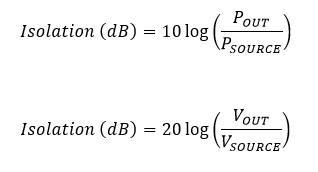# Switching Considerations for RF and Microwave Signals

## Overview

RF and microwave signals require extra consideration when choosing switching hardware to preserve signal integrity and protect RF test equipment. The following sections discuss common switching considerations for RF and microwave systems, including bandwidth, insertion loss, voltage standing wave ratio (VSWR), isolation, crosstalk, and rise time.

## Specifications Dependent on Signal Frequency

It is important to note that RF switch specifications are dependent upon the signal frequency. For example, the PXI-2796 Dual 6x1 multiplexer is rated for 40 GHz, but it has different specs based on signal frequency. Therefore, you should always look for the specifications closest to your application frequency.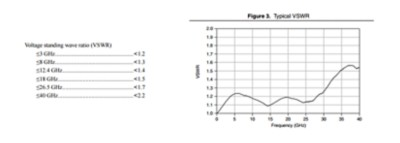Figure 1. Both guaranteed VSWR (left) and typical VSWR (right) specs vary based on signal frequency.

## Characteristic Impedance

Characteristic impedance is a transmission line parameter that determines how propagating signals are transmitted or reflected in the line. The following equation and figure represent the components of characteristic impedance.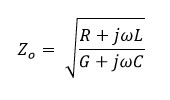Where:

Z0 is the characteristic impedance

L is the inductance per length

C is the capacitance per length

R is the resistance per length

G is the dielectric conductance per length

ω is the frequency (radians/s)

If a transmission line is in the system, its characteristic impedance must also match the source and the load for maximum power transfer. In an ideal, lossless transmission line, there is no series resistance (R = 0) or dielectric loss (G = 0). This means that the characteristic impedance is independent from frequency.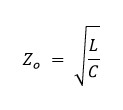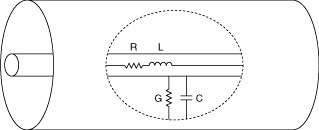Figure 2. Detailed View of Transmission Line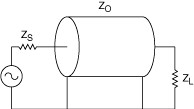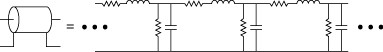Figure 3. Impact of Transmission Line on Example Circuit

Maximum power is transferred in a system from the source to the load when both have the same impedance.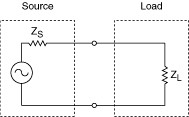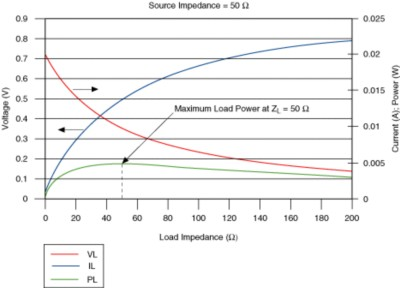Figure 4. Match Characteristic Impedance Across Source, Transmission Lines, and Load to Ensure Signal Integrity

## Insertion Loss

As a high-frequency signal traverses through a switch module, it is attenuated by series resistance, dielectric absorption, and by reflections from impedance mismatches. This attenuation is called insertion loss. The amount of signal remaining at the output of the switch module is represented as a ratio to the input signal and given in deciBels (dB):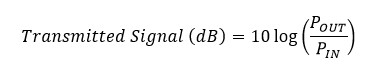Where POUT is the output power level, and PIN is the input power level. This ratio can also be stated in terms of signal voltage:Because the switch attenuates the signal, output magnitude is less than input magnitude, and the transmitted signal ratio is always <0 dB. By convention, insertion loss is defined as the ratio of input signal to the output signal, and is given in decibels (dB):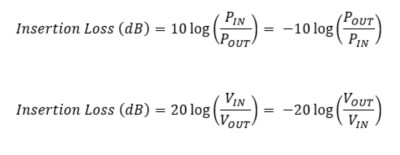Because output magnitude is less than input magnitude, insertion loss is >0 dB. The contributing factors of series resistance, dielectric loss, and mismatch reflections are frequency dependent and generally result in insertion loss rising with signal frequency.

Complex RF Switching Architectures

## VSWR

Any impedance mismatches along a transmission line causes partial reflection of the propagating signals. The difference in impedance determines the magnitude of the reflection. The length of a mismatched section determines the lowest signal frequencies that will reflect off of the section. Voltage standing wave ratio (VSWR) is a measure of signal reflection.

With an incident sine wave into the switch module, some of the signal reflects back down the line. The reflected wave interferes with the incident wave. VSWR is the ratio of maximum to minimum amplitude in the resulting interference wave and is represented by the following formula: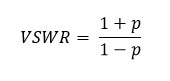Where p is the reflection coefficient. Reflections can also be represented as a logarithmic ratio of the reflected signal to the input signal. This is called return loss: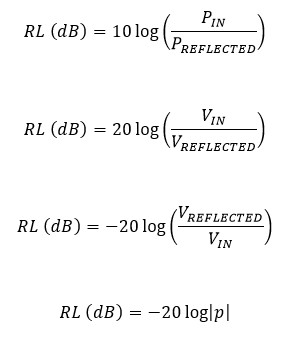Complex RF Switching Architectures

## Rise Time

Given an ideal step input, rise time is the time required for the output signal voltage to rise from 10% to 90% of the step amplitude.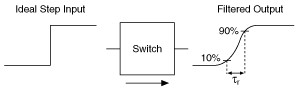Figure 5. Switching Can Effect the Rise Time of Square Edges

Rise time can be related to bandwidth with the approximation: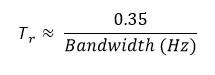## Bandwidth

Signal bandwidth is the useful range of signal frequencies for a switch. NI switch modules are specified for frequencies down to DC so bandwidth is specified as the maximum recommended signal frequency. In many cases, this is the highest signal frequency that the switch can maintain <3 dB of insertion loss. Some RF modules specify bandwidths based on reflections instead of insertion loss. Refer to VSWR.

If a signal is purely sinusoidal, the bandwidth/insertion loss rating of a switch module can be directly applied. For signals that have multiple frequency components, like a square wave, the rating may be difficult to apply.

A square wave can be represented using a natural harmonic series, where only the odd harmonics are used. The frequency components that make up an ideal square wave are infinite, as shown below: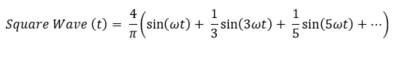Where ω is frequency in radians per second.

A square wave may have a fundamental frequency below the rated bandwidth. However, harmonics above the rating may be attenuated by increasing insertion loss. For example, consider a 2 V peak-to-peak square wave. The square wave can be approximated by the summation of multiple harmonic sine waves. Three harmonics are shown in the following figure: the fundamental frequency (or first harmonic), the fifth, and the 13th harmonic.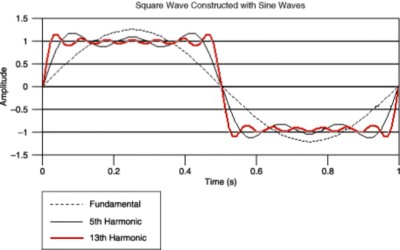Figure 6. Square Waves Represented by Sine Waves of Varying Frequencies

If the frequency content of a square wave is known, the required switch bandwidth can be determined based on the highest harmonic to be passed with minimal distortion. If the frequency content is unknown, the -3 dB point can be approximated using the rise time of the harmonic to be preserved. The rise time is related to the -3 dB point bandwidth by the approximation: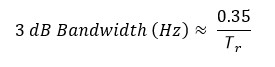Where τ is the rise time from 10% to 90% of the signal amplitude. Using the fifth harmonic of the square wave from the previous example: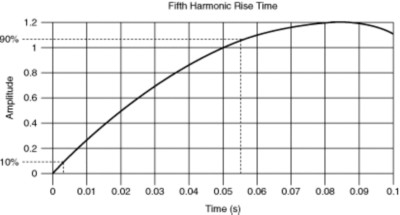Figure 7. Rise Time of Fifth Harmonic

Where τ = 0.0515 s.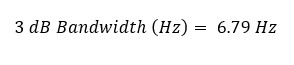To preserve the rise time of the signal, a switch with an insertion loss of 3dB at 6.79 Hz minimum should be chosen. For a square wave, once the 5th or 7th harmonic is reached, the change in the rise time is minimal. In the previous example a switch with an insertion loss of 3 dB at 7 Hz will be sufficient to pass as a 1 Hz square wave. The rule of thumb is to choose a switch for square wave signals with a -3 dB point at a frequency 7 times the fundamental frequency of the square wave. If less attenuation is desired, the -3 dB point should be a higher frequency.

## Crosstalk

Crosstalk is the amount of signal from one active channel that appears on another active channel. Both channels are assumed to be appropriately terminated. Crosstalk is specified in dB of transmission: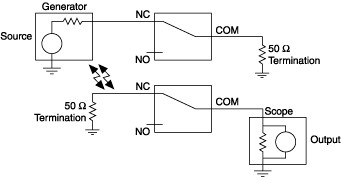Figure 8. Crosstalk on a General Purpose Switch Module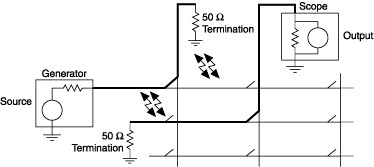Figure 9. Crosstalk on a Matrix Switch Module

Crosstalk is specified in dB of transmission, and calculated according to the following formulas: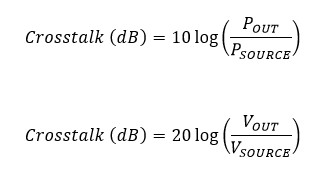Complex RF Switching Architectures

## Isolation

Isolation is the ability to keep a signal on an unused channel from appearing on an active, terminated channel. Isolation is specified in dB of rejection: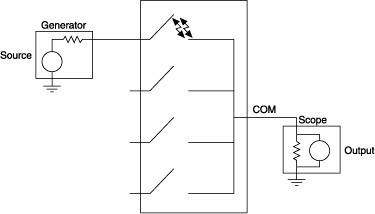Figure 10. Open Relay Isolation on a Multiplexer Switch Module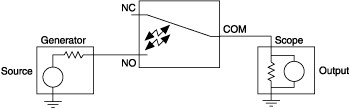Figure 11. Open Relay Isolation on a General Purpose Switch Module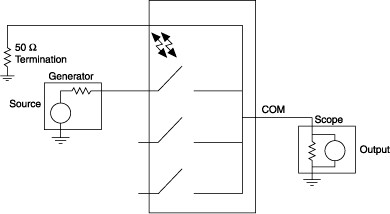Figure 11. Channel-to-Channel Isolation on a Multiplexer Switch Module

Isolation is specified in dB of rejection, according to either one of the following formulas: